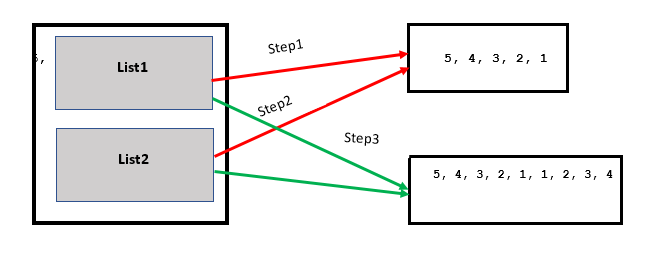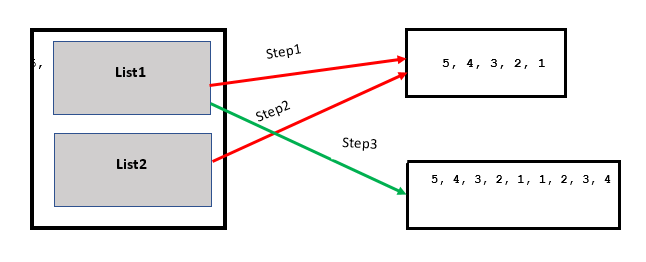Open In App

# Python | a += b is not always a = a + b

In python a += b doesn’t always behave the same way as a = a + b, the same operands may give different results under different conditions. But to understand why they show different behaviors you have to deep dive into the working of variables.

So first, you need to know what happens behinds the scene.

Creating New Variable:

## Python3

 `a ``=` `10``print``(``" id of a : "``, ``id``(``10``) ,``" Value : "``, a  )`

Output :

`id of a :  11094592  Value :  10`

Here in the above example, value 10 gets stored in memory and its reference gets assigned to a.

Modifying The Variable:

## Python3

 `a ``=` `10`  `# Assigning value to variable creates new object``print``(``" id of a : "``, ``id``(a) ,``" Value : "``, a  )` `a ``=` `a ``+` `10` `# Modifying value of variable creates new object``print``(``" id of a : "``, ``id``(a) ,``" Value : "``, a  )``  ` `a ``+``=` `10` `# Modifying value of variable creates new object``print``(``" id of a : "``, ``id``(a) ,``" Value : "``, a  )`

Output :

```id of a :  11094592  Value :  10
id of a :  11094912  Value :  20
id of a :  11095232  Value :  30```

As whenever we create or modify int, float, char, string they create new objects and assign their newly created reference to their respective variables.

But the same behavior is not seen in the list

## Python3

 `a ``=` `[``0``, ``1``] ``# stores this array in memory and assign its reference to a``print``(``"id of a: "``,``id``(a) , ``"Value : "``, a )` `a ``=` `a ``+` `[``2``, ``3``] ``# this will also behave same store data in memory and assign ref. to variable``print``(``"id of a: "``,``id``(a) , ``"Value : "``, a )` `a ``+``=` `[``4``, ``5``]``print``(``"id of a: "``,``id``(a) , ``"Value : "``, a )` `#But now this will now create new ref. instead this will modify the current object so``# all the other variable pointing to a will also gets changes`

Output:

```id of a:  140266311673864 Value :  [0, 1]
id of a:  140266311673608 Value :  [0, 1, 2, 3]
id of a:  140266311673608 Value :  [0, 1, 2, 3, 4, 5]  ```

At this point you can see the reason why a = a + b some times different from a += b.

Consider these examples for list manipulation:
Example 1:

## Python3

 `list1 ``=` `[``5``, ``4``, ``3``, ``2``, ``1``]``list2 ``=` `list1``list1 ``+``=` `[``1``, ``2``, ``3``, ``4``] ``# modifying value in current reference` `print``(list1)``print``(list2) ``# as on line 4 it modify the value without creating new object``             ``# variable list2 which is pointing to list1 gets changes`

Output:

```[5, 4, 3, 2, 1, 1, 2, 3, 4]
[5, 4, 3, 2, 1, 1, 2, 3, 4]```Example 2

## Python3

 `list1 ``=` `[``5``, ``4``, ``3``, ``2``, ``1``]``list2 ``=` `list1``list1 ``=` `list1 ``+` `[``1``, ``2``, ``3``, ``4``]` `# Contents of list1 are same as above``# program, but contents of list2 are``# different.``print``(list1)``print``(list2)`

Output:

```[5, 4, 3, 2, 1, 1, 2, 3, 4]
[5, 4, 3, 2, 1]```• expression list1 += [1, 2, 3, 4] modifies the list in-place, which means it extends the list such that “list1” and “list2” still have the reference to the same list.
• expression list1 = list1 + [1, 2, 3, 4] creates a new list and changes “list1” reference to that new list and “list2” still refer to the old list.